• EL表达式保留小数点位数
2020-06-16 00:26:20
                    <td><fmt:formatNumber type="number" value="${orderitem.product.price*orderitem.num}" maxFractionDigits="2" pattern="0.00"/></td>  首先要导入标签块 idea自动生成即可 maxFractionDigits=“2” pattern="0.00"代表位数和格式 pattern不加就报错 也不知道为啥 先用了再说吧 有知道的欢迎留言 巨弱流泪 更多相关内容 • python保留小数点位数的方法：首先新建py文件，输入【a=('%.2f' % a)】即可保留2位小数；然后如果输入【a=('%.4f' % a)】，就保留4位小数；最后也可以输入【a=format(a, '.2f')】来保留小数点位数。本教程操作环境：... python保留小数点位数的方法：首先新建py文件，输入【a=('%.2f' % a)】即可保留2位小数；然后如果输入【a=('%.4f' % a)】，就保留4位小数；最后也可以输入【a=format(a, '.2f')】来保留小数点位数。本教程操作环境：windows7系统、pycharm2020版，DELL G3电脑。 python保留小数点位数的方法： 第一步、打开pycharm，新建一个py文件，输入a=1.2222345 a=('%.2f' % a) print(a) 代码，如下图所示：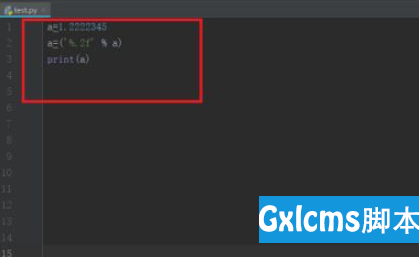第二步、运行py文件之后，可以看到a小数点保留2位小数了，如下图所示：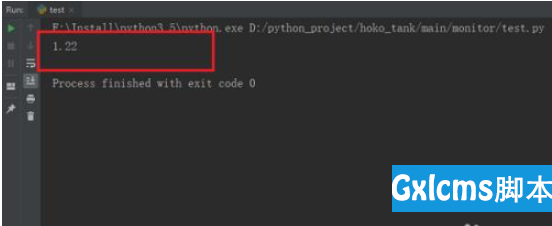第三步、如果输入a=('%.4f' % a)，就保留4位小数，需要注意是四舍五入，如下图所示：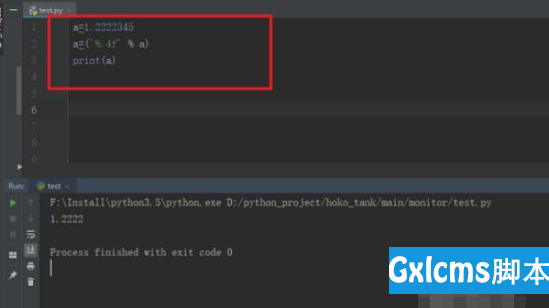第四步、我们也可以输入a=format(a, '.2f')来保留小数点位数，如下图所示：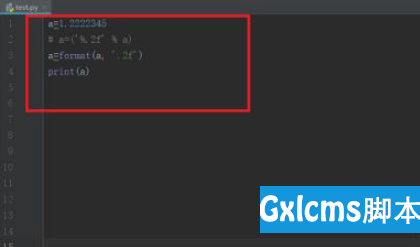第五步、运行py文件，可以看到保留2位小数，同样也是四舍五入，如下图所示：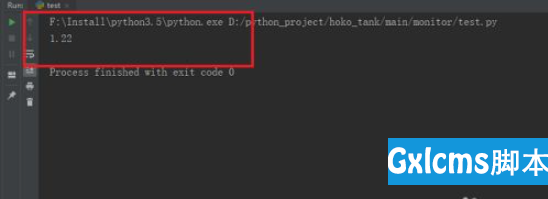相关免费学习推荐：python视频教程 展开全文• 保留两位小数 方法一： { double c = 3.154215; java.text.DecimalFormat myformat=new java.text.DecimalFormat("0.00"); String str = myformat.format(c); } 方式二： { java.text.DecimalFormat df = ... ## 保留两位小数 方法一： { double c = 3.154215; java.text.DecimalFormat myformat=new java.text.DecimalFormat("0.00"); String str = myformat.format(c); } 方式二： { java.text.DecimalFormat df = new java.text.DecimalFormat("#.00"); df.format(你要格式化的数字); } 例：new java.text.DecimalFormat(“#.00”).format(3.1415926) .00 表示两位小数 #.0000四位小数 以此类推… 方式三： { double d = 3.1415926; String result = String .format("%.2f"); } %.2f %. 表示 小数点前任意位数 2 表示两位小数 格式后的结果为f 表示浮点型 方法四（四舍五入）： { double f = 111231.5585; BigDecimal b = new BigDecimal(f); //保留2位小数 double f1 = b.setScale(2,BigDecimal.ROUND_HALF_UP).doubleValue(); } ## 小数点后补0 如果float num = 3.14f; 现在需要保留5为小数。这里要知道一点，不管是Float类型还是Double类型，他们都是不会记住小数位数的，而BigDecimal就可以记住。而且这种需求我们肯定是用String来记录，因为在UI显示肯定是要转换为String类型的。 { float num = 3.140f; BigDecimal b = new BigDecimal(num); //保留5位并且属于四舍五入类型，当然这里的四舍五入没有任何意义，可以选择其他类型。 b.setScale(5, BigDecimal.ROUND_HALF_UP).toString(); } 展开全文android java apache • 这两个浮点数相除时，由于除不尽，而又没有设置精度和保留小数点位数，导致抛出异常。而第二个例子中0.6和0.4是字符串类型，由于BigDecimal存储特性，通过BigInteger记录BigDecimal的值，所以，0.6和0.4可以非常正确... 转载自逸学堂BigDecimal 的那些坑事儿 最近查看rebate数据时，发现一个bug，主要现象是，当扣款支付宝的账号款项时，返回的是数字的金额为元，而数据库把金额存储为分，这中间要做元与分的转化，这个转化规则很简单，就是*100的，所以一开始代码很简单，如下。 Float f = Float.valueOf(s); f =f*100; Long result = f.longValue();  当s=”9.86”时，杯具出现了，result的结果为985而不是986，float的精度损失导致float(985.99994)转化为整形时，丢掉小数部分成为985，简单的方法，我们可以提高精度使用双精度的double类型，提高精度，比如 Double d = Double.valueOf(s); d = d*100; Long result = d.longValue();  当s=”9.86”时，确实能够得到正确结果，但是当s=”1219.86”时，这时候由于精度问题导致最终的result为121985为不是121986。当时以为使用double解决的问题，其实隐藏更隐蔽的bug。 Double d = Double.valueOf(s); d = d*100+0.5;// 注意这里，我们使用的是+0.5的形式。 Long result = d.longValue();  但是，我们使用的java语言，java应该有更优雅的解决方案，那就是BigDecimal。 使用BigDecimal的解决方案成这个样子 Double dd= Double.valueOf(s); BigDecimal bigD = new BigDecimal(dd); bigD = bigD.multiply(new BigDecimal(100)); Long result = bigD.longValue();  狂晕，输出结果是985为不是986，打印bigD System.out.println(bigD.toString()); 输出：985.9999999999999431565811391919851303100585937500  不会再加上一个BigDecimal(0.5)吧。我相信在使用过BigDecimal过程中，肯定有那里不对的地方，multiply方法中可以传入精度，那就构造MathContext对象，修改如下。 Double dd= Double.valueOf(s); BigDecimal bigD = new BigDecimal(dd); MathContextmc = new MathContext(4,RoundingMode.HALF_UP); //4表示取四位有效数字，RoundingMode.HALF_UP表示四舍五入 bigD= bigD.multiply(new BigDecimal(100),mc); Long result = bigD.longValue();  最后结果输出为986，貌似已经找到完成解决方案，其实不然，注意到MathContext中的4了嘛？这是因为我们保留4位有效数字，假如我们输入的数字是大于4的，比如1219.86，最终输出结果是122000，这是因为1219.86保留4位有效数字时，第四位的9四舍五入，除去精确位补零，所以最终结果成了122000。问题就成了，我们必须知道元变分后的最终有效位数，”9.86”，有效位数是4，”19.86”有效位数是5，把字符串s的长度传过去就可以了，那么代码如下 Double dd =Double.valueOf(s); BigDecimalbigD = new BigDecimal(dd); MathContextmc = new MathContext(s.length(),RoundingMode.HALF_UP); //4表示取四位有效数字，RoundingMode.HALF_UP表示四舍五入 bigD= bigD.multiply(new BigDecimal(100),mc); Long result = bigD.longValue();  1public BigDecimal(double val)将double表示形式转换为BigDecimal 2public BigDecimal(int val)将int表示形式转换为BigDecimal 3public BigDecimal(String val)将字符串表示形式转换为BigDecimal 通过这三个构造函数，可以把double类型，int类型，String类型构造为BigDecimal对象，在BigDecimal对象内通过BigIntegerintVal存储传递对象数字部分，通过int scale;记录小数点位数，通过int precision;记录有效位数（默认为0）。 BigDecimal的加减乘除就成了BigInteger与BigInteger之间的加减乘除，浮点数的计算也转化为整形的计算，可以大大提供性能，并且通过BigInteger可以保存大数字，从而实现真正大十进制的计算，在整个计算过程中，还涉及scale的判断和precision判断从而确定最终输出结果。 我们先看一个例子 BigDecimal d1 = new BigDecimal(0.6); BigDecimal d2 = new BigDecimal(0.4); BigDecimal d3 = d1.divide(d2); System.out.println(d3);  大家猜一下，以上输出结果是？再接着看下面的代码 BigDecimal d1 = new BigDecimal(“0.6”); BigDecimal d2 = new BigDecimal(“0.4”); BigDecimal d3 = d1.divide(d2); System.out.println(d3);  看似相似的代码，其结果完全不同，第一个例子中，抛出异常。第二个例子中，输出打印结果为1.5。造成这种差异的主要原因是第一个例子中的创建BigDecimal时，0.6和0.4是浮动类型的，浮点型放入BigDecimal内，其存储值为 0.59999999999999997779553950749686919152736663818359375 0.40000000000000002220446049250313080847263336181640625  这两个浮点数相除时，由于除不尽，而又没有设置精度和保留小数点位数，导致抛出异常。而第二个例子中0.6和0.4是字符串类型，由于BigDecimal存储特性，通过BigInteger记录BigDecimal的值，所以，0.6和0.4可以非常正确的记录为 0.6 0.4  两者相除得出1.5来。 对于第一个例子，如果我们想得到正确结果，可以这样来 BigDecimal d1 = new BigDecimal(0.6); BigDecimal d2 = new BigDecimal(0.4); BigDecimal d3 = d1.divide(d2, 1, BigDecimal.ROUND_HALF_UP);  现在看我们留下的那个问题，使用更优雅的方式解决元转化为分的方式，上一个问题中，我们通过传递s.length()从而获得精度，如果之前的s是double类型的，那边这样的方式就会有问题，通过上面的例子，我们可以调整为一下的通用方式 Double dd= Double.valueOf(s); BigDecimal bigD = new BigDecimal(dd); bigD = bigD.multiply(newBigDecimal(100)). divide(1, 1, BigDecimal.ROUND_HALF_UP); Long result = bigD.longValue();  我们通过/1，然后设置保留小数点方式，以及设置数字保留模式，从而得到两个数乘积的小数部分。还有以下模式 枚举常量摘要 ROUND_CEILING 向正无限大方向舍入的舍入模式。 ROUND_DOWN 向零方向舍入的舍入模式。 ROUND_FLOOR 向负无限大方向舍入的舍入模式。 ROUND_HALF_DOWN 向最接近数字方向舍入的舍入模式，如果与两个相邻数字的距离相等，则向下舍入。 ROUND_HALF_EVEN 向最接近数字方向舍入的舍入模式，如果与两个相邻数字的距离相等，则向相邻的偶数舍入。 ROUND_HALF_UP 向最接近数字方向舍入的舍入模式，如果与两个相邻数字的距离相等，则向上舍入。 ROUND_UNNECESSARY 用于断言请求的操作具有精确结果的舍入模式，因此不需要舍入。（默认模式） ROUND_UP 远离零方向舍入的舍入模式。  总结： 1：尽量避免传递double类型，有可能话，尽量使用int和String类型。 2：做乘除计算时，一定要设置精度和保留小数点位数。 3：BigDecimal计算时，单独放到try catch内。 展开全文BigDecimal • 其中A是要保留的原数字，B是要保留位数。 比如：3.1415926保留2位就是： Number(3.1415926).toFixed(2); 测试demo: <!DOCTYPE html> <html> <head> <meta charset="utf-8"> <...JavaScript • char placesbuffer = { 0 }; char* decimal_places(char*str,int i) { memset(placesbuffer, 0, 32); char* decimal_places = strchr(str, '.'); if (decimal_places) { int index = (decimal_places + (i... • ## C# 保留小数点位数 千次阅读 2020-07-23 11:10:53 说明：保留小数点后面的位数是按 .号后面0的个数计算的。 实例： decimal num=2.1; string strNum=num.ToString("#0.00");//保留两位->2.10 2、ToString("FN") 说明：N代表保留的小数点位数，F不区分大小写 ... • 例如: 保留小数点后1位，则 n = 10 * n= 1 / 0.1 保留小数点后2位，则 n = 100 * n= 1 / 0.01 保留小数点后3位，则 n = 1000 * n= 1 / 0.001 至于为什么是先乘后除，可以参考如下文章： ... • 1、当前方式是保留两位小数点，并且四舍五入 BigDecimal b = new BigDecimal(546542.1214); double discount = b.setScale(2, BigDecimal.ROUND_HALF_UP).doubleValue(); 2、当前方式是直接保留两位小数点 ...java java-ee • java保留两位小数问题：方式一：四舍五入doublef=111231.5585;BigDecimalb=newBigDecimal(f);doublef1=b.setScale(2,BigDecimal.ROUND_HALF_UP).doubleValue();保留两位小数---------------------------------------... • Integer计算保留小数点位数 使用bigDecimal.setScale BigDecimal bigDecimal = BigDecimal.valueOf((float) 2 / 3); double ratio = bigDecimal.setScale(2, RoundingMode.HALF_UP).doubleValue(); 使用...java • 金额保留两位小数，积分取整数 <small style="padding-bottom: 5%">订单应付金额</small>${pointsOrders.ordersAmount?string("0.00")}元<br/> <small style="padding-top: 5%">订单应付...freemarker
• 前面的数，表示了即将输出的这个数占多少位数， .**后面的数，表示了即将输出的这个数将保留多少位小数 同时，如果 . 前面的数是一个正数，则说明它是向右对齐的，即补位补在前面，如果是负数，则相反。
• 前面的数，表示了即将输出的这个数占多少位数，.**后面的数，表示了即将输出的这个数将保留多少位小数 同时，如果 . 前面的数是一个正数，则说明它是向右对齐的，即补位补在前面，如果是负数，则相反。
• 浮点数，根据精度保留小数点位数，实际值不够的显示0{a:"",auccuracy:""} 整数，需要加上逗号{a:"",real_type:'11'} */ let data1={value:"123,432.1",accuracy:'1'} ...
• C#中如何只保留小数点后面两位方法： 1、num.ToString("#0.00"); //点后面几个0就保留几位 double num=0.121245；string result=num.ToString("#0.00"); //点后面几个0就保留几位Console.WriteLine(result)。 2、...C#
• 保留小数点位数 //保留小数点位数 function retainDecimalDigit(var value,var digitNum){ if (value != 0 && (value == "" || value == null || value == undefined)) { return ""; } return (value...
• 1.matlab大段注释的方法 方法一： 注释语句的快捷键是Ctrl+R 取消注释的快捷键是Ctrl+T 并且支持一次注释（或者取消注释）多行语句。...2.保留小数点位数 https://blog.csdn.net/xiakexiaohu/ar...matlab 安装 笔记 函数
• toFixed [MDN]...一、保留小数点后两位四舍五入 export function NumFilter (value) { // 截取当前数据到小数点后两位 let realVal = parseFloat(value).toFixed(2)yarn 前端 javascript
• 一、保留小数点后两位四舍五入 export function NumFilter (value) { // 截取当前数据到小数点后两位 let realVal = parseFloat(value).toFixed(2) return realVal } 二、保留两位小数不四舍五入 export ...javascript 前端 vue.js
• 有时候我们在时候java的时候需要保留小数点位数，那么你知道java代码如何保留小数点位数吗？那么下面就让爱站小编为大家介绍java代码保留小数点位数实现方法。import java.text.* ;import java.math.* ;public...
•C#
• Python：使用f-string保留小数点位数 格式 f"{num:xxx}" 其中xxx的格式如下 格式 说明 width 整数width指定宽度 0width 整数width指定宽度，0表示最高位用0补足宽度 width.precision 整数width指定...
• Log.d("小数点位数","format: "+format+" format2: "+format2+" format3: "+format3+" format4: "+format4+" format5: "+format5+" format6: "+format6); 打印信息: 2020-08-04 18:07:44.468 30949-30949/...android
• 保留七位小数点（简概代码） import java.text.DecimalFormat; double s=r*r; DecimalFormat df=new DecimalFormat("#.0000000"); System.out.println(df.format(s)); 转载于:...
• 　举个例子，假如我们需要保留两位小数，我们可以这样写 　DecimalFormat df = new DecimalFormat(0.00); 　测试如下： 　double d = 0.200; 　DecimalFormat df = new DecimalFormat(0.00); 　System.out....
• 在C++中有大致两种保留方式 其一是cin>> cout>> 另一种是scanf()与printf() 第二种相较于第一种保留小数点后位置时更简便 如：一： #include using namespace std; int main() {double a=1.234; cout;} *注意：若a...c++ 开发语言...